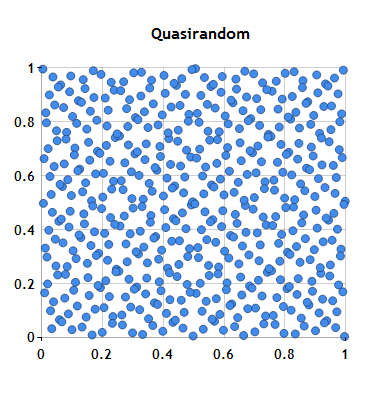# Quasirandom Points

A quasirandom sequence is a set of n-tuples that fills n-space more uniformly than uncorrelated random points. NMath provides both Sobol and Niederreiter quasirandom number generators for .NET applications.

For example, this C# code creates a Niederreiter quasirandom number generator object to generate quasirandom vectors of length 2:

```int dim = 2;
NiederreiterQuasiRandomGenerator nqrg =
new NiederreiterQuasiRandomGenerator(dim);```

You can use an NMath quasirandom generator to fill a matrix or array. The points are the columns of the matrix, so the number of rows in the given matrix must be equal to the dimension of the quasirandom number generator. Here we create and fill a 2 x 500 matrix with quasirandom numbers from a uniform (0,1) distribution.

```int n = 500;
DoubleMatrix A = new DoubleMatrix(dim, n);
nqrg.Fill(A);```

We can plot the points to see the distribution.Let’s compare the results above with the distribution from a standard pseudorandom generator.

```int seed = 0x345;
RandomNumberStream stream = new RandomNumberStream( seed, RandomNumberStream.BasicRandGenType.MersenneTwister );
DoubleRandomUniformDistribution unifDist = new DoubleRandomUniformDistribution();
DoubleMatrix B = new DoubleMatrix( dim, n, stream, unifDist );```

Plotting the results shows that the pseudorandom points are much less uniformly distributed. Regions of higher and lower density are clearly evident.Quasirandom numbers have many applications, especially in Monte Carlo simulations. For instance, a classic use of the Monte Carlo method is for the evaluation of definite integrals. Let’s use an NMath Sobol generator to approximate the integral of a function F over a 6-dimensional unit cube. The function is defined by

F(x) = 1*cos(1*x1) * 2*cos(2*x2) * 3*cos(3*x3) *…* 6*cos(6*x6)

where x = (x1, x2,…, x6) is a point in 6-dimensional space.

This is the implementation of the function F:

```static double F( DoubleVector x )
{
double y = 1;
for ( int i = 1; i <= x.Length; i++ )
{
y *= i * Math.Cos( i * x[i - 1] );
}
return y;
}```

In the following C# code we provide our own primitive polynomials for initializing the quasirandom generator. Primitive polynomials have coefficients in {0, 1} and are specified by BitArray’s containing the polynomial coefficients with the leading coefficient at index 0. We can supply either dim polynomials, or dim – 1 polynomials. If we specify dim – 1 polynomials, the primitive polynomial for the first dimension will be initialized with a default value.

```dim = 6;

BitArray[] primitivePolys = new BitArray[dim];
primitivePolys = new BitArray( new bool[] { true, true } ); // x + 1
primitivePolys = new BitArray( new bool[] { true, true, true } ); // x^2 + x + 1
primitivePolys = new BitArray( new bool[] { true, false, true, true } ); // x^3 + x + 1
primitivePolys = new BitArray( new bool[] { true, true, false, true } ); // x^3 + x^2 + 1
primitivePolys = new BitArray( new bool[] { true, false, false, true, true } ); // x^4 + x + 1
primitivePolys = new BitArray( new bool[] { true, true, false, false, true } ); // x^4 + x^3 + 1

SobolQuasiRandomGenerator sobol = new SobolQuasiRandomGenerator( dim, primitivePolys );```

Now let’s use this generator to approximate the integral:

```int numPoints = 180000;
DoubleMatrix points = new DoubleMatrix( dim, numPoints );
sobol.Fill( points );

double sum = 0;
for ( int i = 0; i < numPoints; i++ )
{
sum += F( points.Col( i ) );
}
sum /= numPoints;

double actualIntegralValue = -0.022;
Console.WriteLine( "Actual integral value = " + actualIntegralValue );
Console.WriteLine( "\nMonte-Carlo approximated integral value = " + sum );```

The output is:

```Actual integral value = -0.022
Monte-Carlo approximated integral value = -0.0232768655843053```

Ken

Top